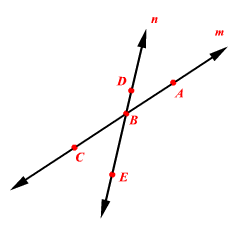# Collinear Points

Three or more points that lie on the same line are collinear points .

Example :The points $A$ , $B$ and $C$ lie on the line $m$ . They are collinear.

The points $D$ , $B$ and $E$ lie on the line $n$ . They are collinear.

There is no line that goes through all three points $A$ , $B$ and $D$ . So, they are not collinear.